# How to Calculate and Solve for Success and Failure | Probability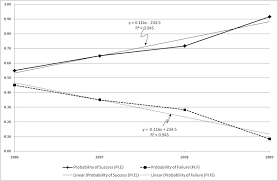The image above represents success and failure.

To compute for success and failure, two essential parameters are needed and these parameters are and N.

The formula for calculating success:

P(success) = x N

Where;

x = Number of successes
N = Total Number of Trials or Items

Let’s solve an example;
Find the success when the x is 12 and N is 14?

This implies that;

x = Number of successes = 12
N = Total Number of Trials or Items = 14

P(success) = x N
P(success) = 12 14
Dividing the numerator and denominator by 2
P(success) = 6 7
P(success) = 0.857

Therefore, the success is 0.857.

The formula for calculating failure:

P(failure) = (N – x) N

Where;

x = Number of successes
N = Total Number of Trials or Items

Let’s solve an example;
Find the failure when the x is 12 and N is 14.

This implies that;

x = Number of successes = 12
N = Total Number of Trials or Items = 14

P(failure) = (N – x) N
P(failure) = (14 – 12) 14
P(failure) = 2 14
Dividing the numerator and denominator by 2
P(failure) = 1 7
P(failure) = 0.142

Therefore, the failure is 0.142.

Nickzom Calculator – The Calculator Encyclopedia is capable of calculating the success and failure.

To get the answer and workings of the success and failure using the Nickzom Calculator – The Calculator Encyclopedia. First, you need to obtain the app.

You can get this app via any of these means:

To get access to the professional version via web, you need to register and subscribe for NGN 1,500 per annum to have utter access to all functionalities.
You can also try the demo version via https://www.nickzom.org/calculator

Apple (Paid) – https://itunes.apple.com/us/app/nickzom-calculator/id1331162702?mt=8
Once, you have obtained the calculator encyclopedia app, proceed to the Calculator Map, then click on Probability under Mathematics.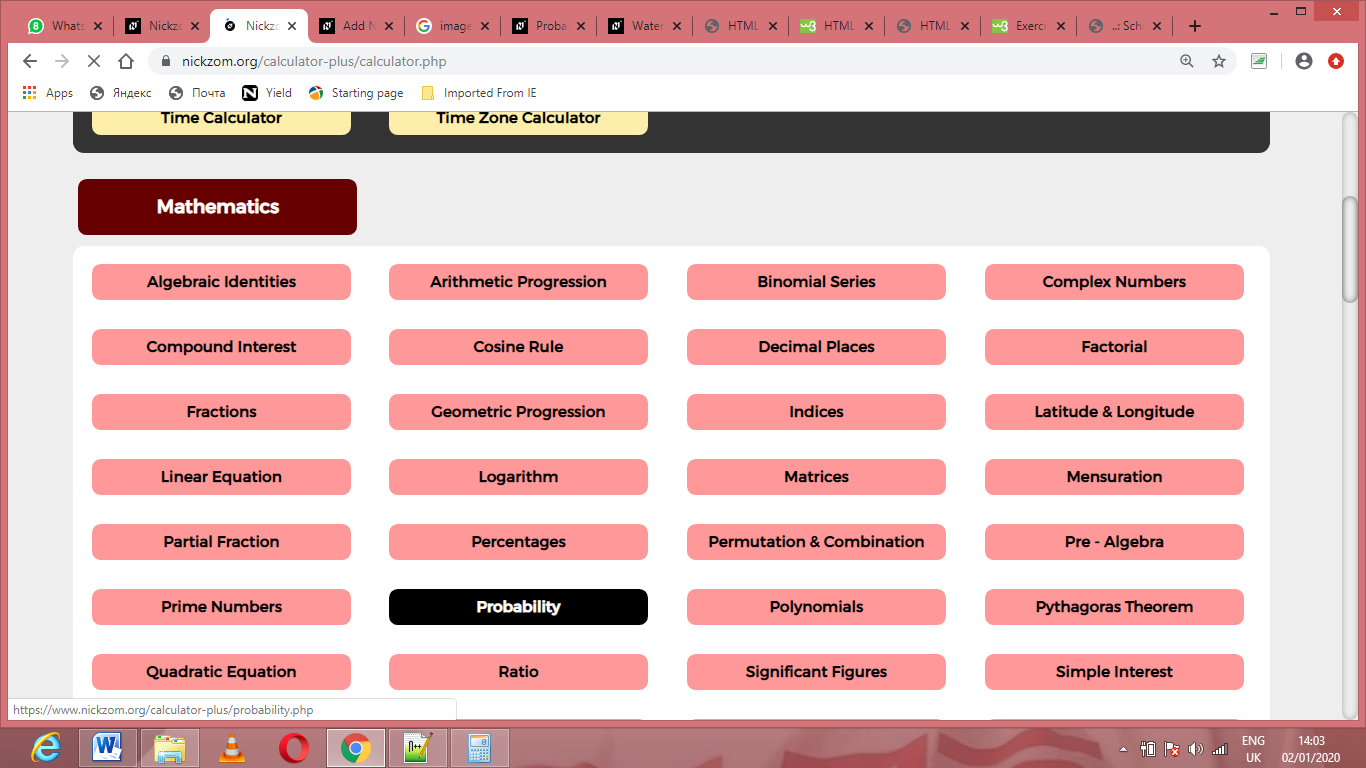Now, Click on Success and Failure under Probability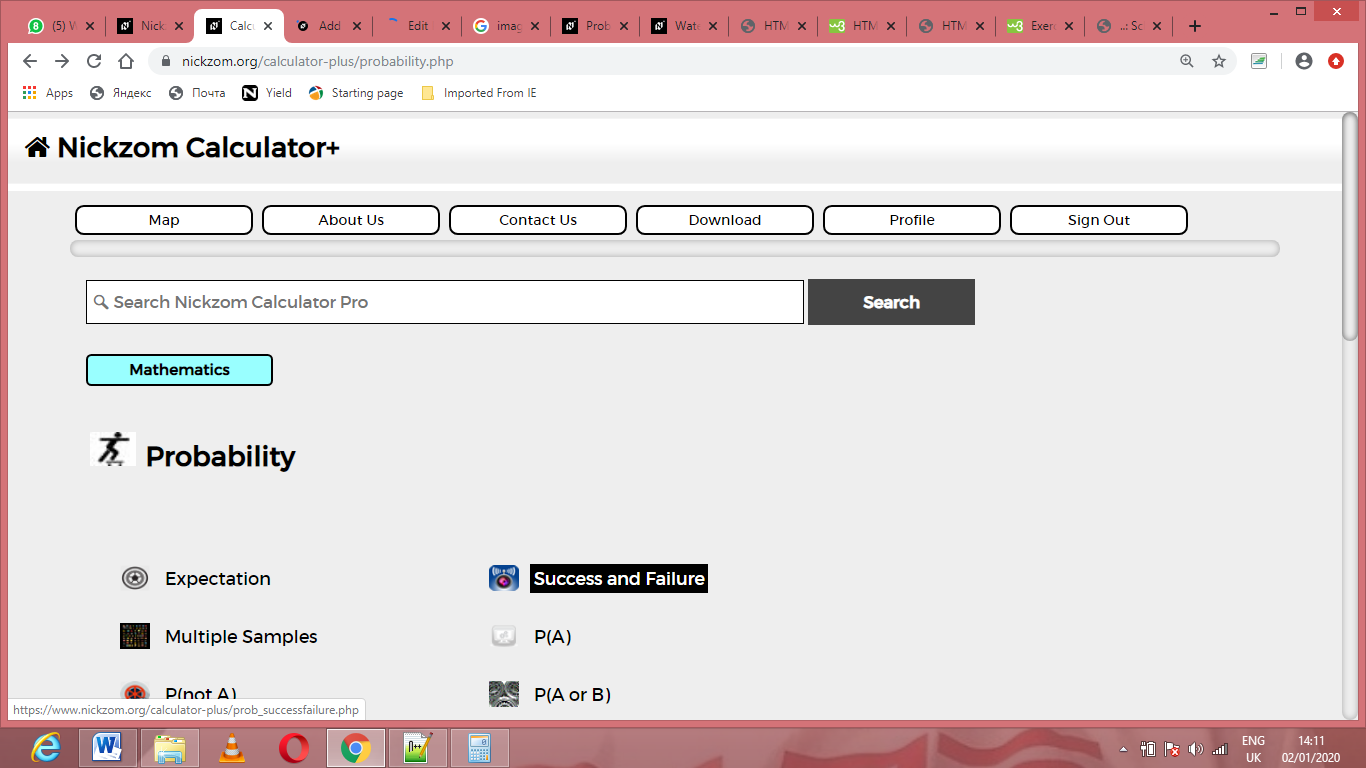The screenshot below displays the page or activity to enter your values, to get the answer for the success and failure according to the respective parameters which are the and N.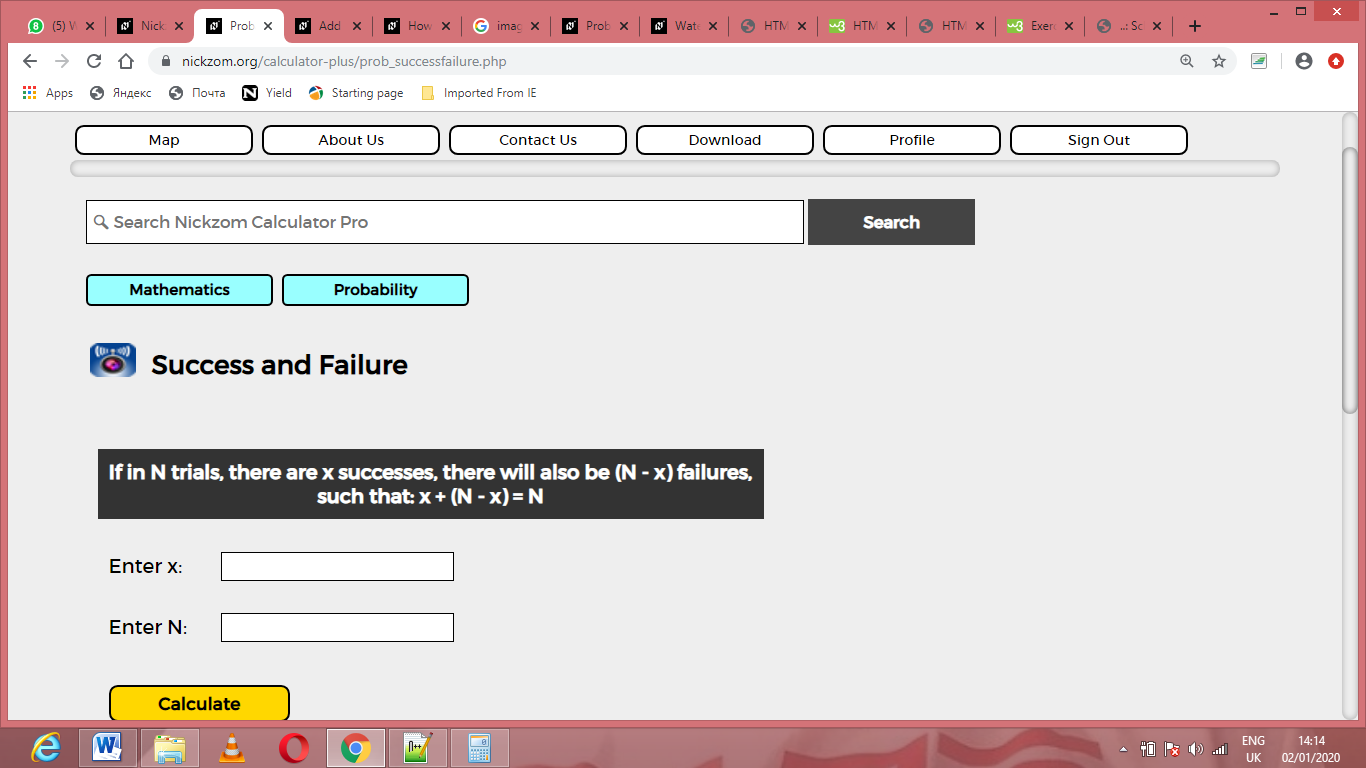Now, enter the values appropriately and accordingly for the parameters as required by the is 12 and is 14.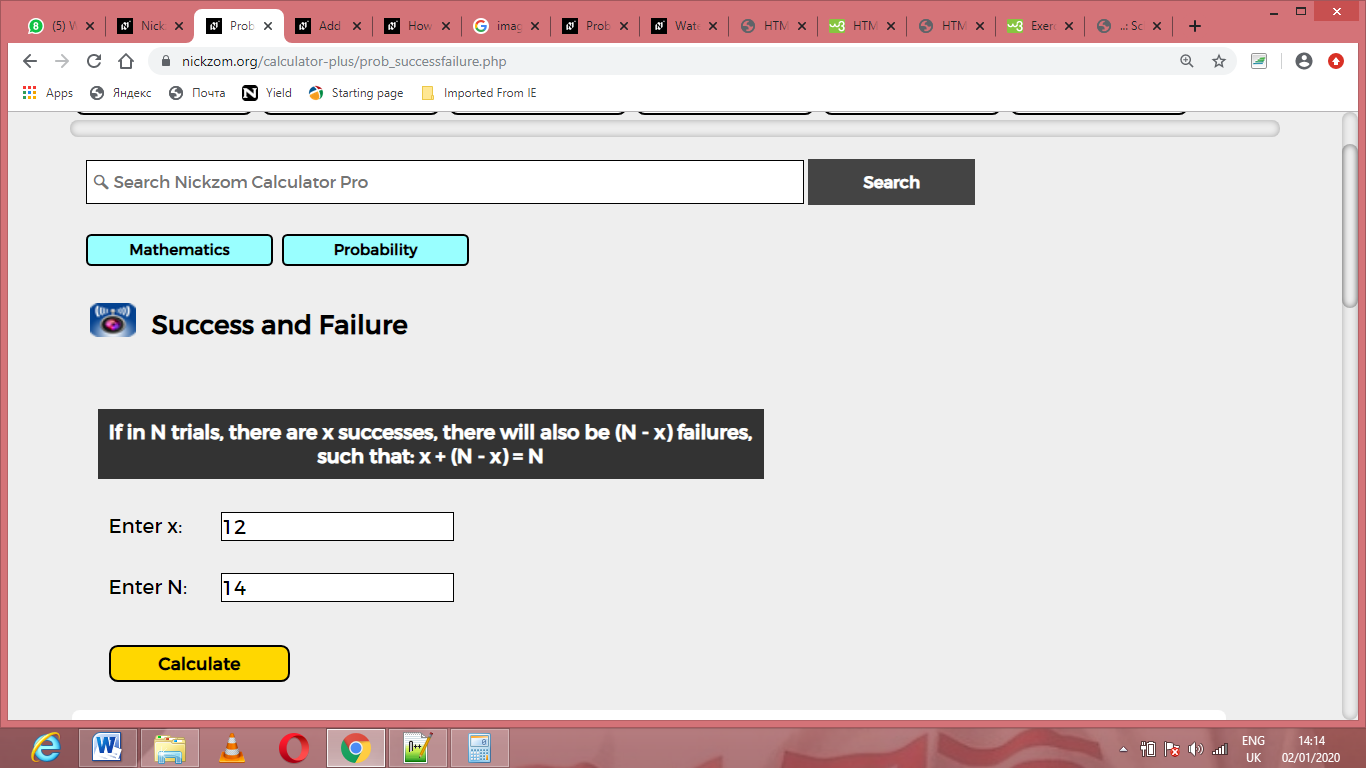Finally, Click on Calculate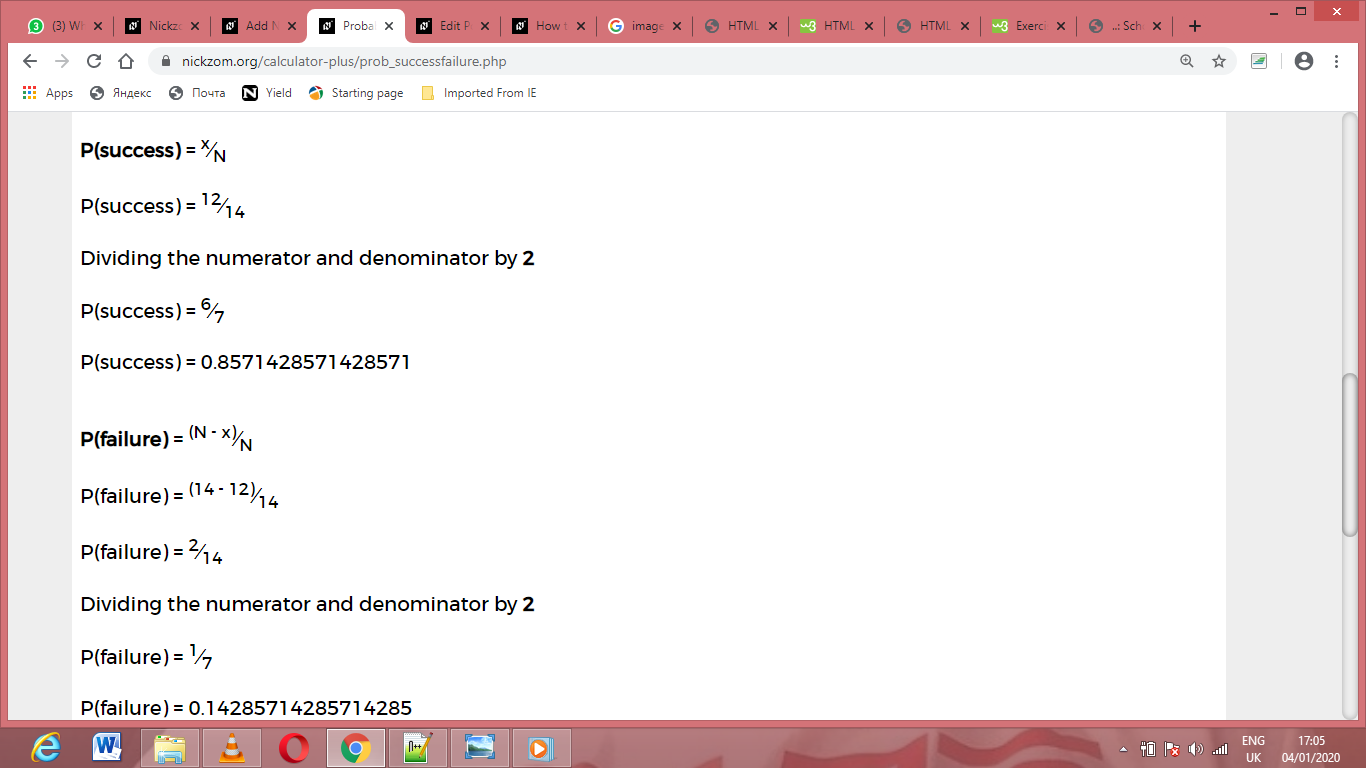As you can see from the screenshot above, Nickzom Calculator– The Calculator Encyclopedia solves for the success and failure, presents the formula, workings and steps too.